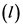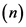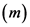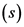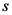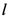# The s orbital is spherical (nondirectional) because

A-the angular wave function of an s orbital is defined by the
quantum number, ell.
B-an s orbital has angular nodes.
C-at a particular distance from the nucleus, the electron
probability is the same in all directions.
D-the angular wave function for all s orbitals is a constant.
E-the angular wave function of an s orbital varies with the
principal quantum number (n).

General guidance

Concepts and reason

This problem is based on the concept of shape of atomic orbitals.
Atomic orbitals are a wave function of an electron. The shape of atomic orbitals is determined by Azimuthal quantum number.

Fundamentals

The position of an electron in an atom is defined by four set of quantum numbers that are principle quantum number that describes the shell number in which the electron is present. Azimuthal quantum numberdepicts the shape of the orbitals, magnetic quantum numberdistinguish the orbitals available within a subshell and spin quantum number depicts the spin of the electron.

Step-by-step

Step 1 of 2

The -orbitals has no nodes and its angular wave function does varies with principle quantum number. Due to is spherical shape, no nodes are possible.

As the distance from the nucleus increases, the density of charge cloud keeps on decreasing. Because with the increase in the distance from the nucleus, the attraction between nucleus and electron decreases.

Consider the properties of -orbitals carefully.

Write the correct statements.

Step 2 of 2

The correct statements are as follows:
A-the angular wave function of an s orbital is defined by the quantum number.
C-at a particular distance from the nucleus, the electron probability is the same in all directions.‎D-the angular wave function for all s orbitals is a constant.

The correct statements are as follows:
A-the angular wave function of an s orbital is defined by the quantum number.
C-at a particular distance from the nucleus, the electron probability is the same in all directions.‎D-the angular wave function for all s orbitals is a constant.

Since, the -orbital is non-directional and spherically symmetrical, the probability of the finding electron is maximum near the nucleus which decreases consistently with the distance. But, due to its spherical symmetry shape, the electron probability is same in all directions.
The angular wave function defines the number of nodes in an orbital. Since, the shape of -orbital is spherical it has zero nodes. So, the angular wave function is constant for all -orbital.

Don’t calculate wrong nodes.

The correct statements are as follows:
A-the angular wave function of an s orbital is defined by the quantum number.
C-at a particular distance from the nucleus, the electron probability is the same in all directions.‎D-the angular wave function for all s orbitals is a constant.